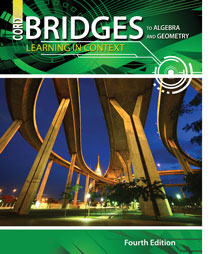# Bridges to Algebra and Geometry - 4th Edition

## Chapter 3: Integers

Some links are repeated for use with more than one lesson.

### The following links apply to the entire chapter.

http://www.math-play.com/Integers-Jeopardy/Integers-Jeopardy.html
NEW This site is a game for practicing integer math operations, for playing alone or with teams.

http://www.explorelearning.com/index.cfm?method=cResource.dspResourcesForCourse&CourseID=211
This site has examples of integer operations and has practice problems for the students to solve.

http://www.aplusmath.com/Flashcards/index.html
This site has practice problems for the students dealing with adding, subtracting, multiplying, and dividing integers.

http://www.math.com/school/subject1/lessons/S1U1L13GL.html
This site gives the rules for properties of integers.

### 3.1 Representing Integers

http://www.math.com/school/subject1/lessons/S1U1L10GL.html
This site defines and shows integers on a number line.

http://www.sosmath.com/algebra/inequalities/ineq02/ineq02.html
This site shows how to graph points and intervals on a number line.

### 3.2 Comparing Integers

http://www.mathsisfun.com/number-line.html
NEW This interactive site demonstrates the inequality comparisons with integers on a number line, with practice problems.

http://www.math.com/school/subject1/lessons/S1U1L11GL.html
This site gives the rules for adding and subtracting integers and has practice problems for the students to solve.

### 3.4 Subtracting Integers

http://www.math.com/school/subject1/lessons/S1U1L11GL.html
This site gives the rules for adding and subtracting integers and has practice problems for the students to solve.

### 3.5 Multiplying and Dividing Integers

http://www.math.com/school/subject1/lessons/S1U1L12GL.html
This site gives the rules for multiplying and dividing integers.

http://www.math.com/school/subject1/lessons/S1U1L12GL.html
This site gives the rules for multiplying and dividing integers.